# Sampling Distribution of the Mean Central Limit Theorem

• Slides: 26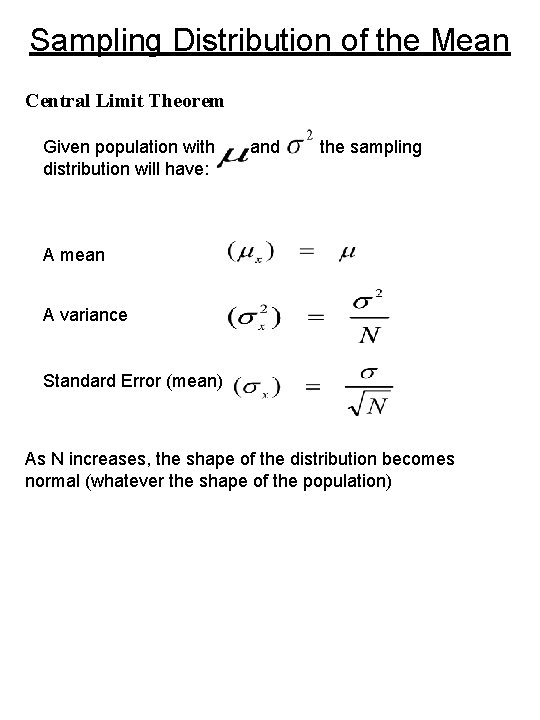Sampling Distribution of the Mean Central Limit Theorem Given population with distribution will have: and the sampling A mean A variance Standard Error (mean) As N increases, the shape of the distribution becomes normal (whatever the shape of the population)Testing Hypothesis Known and Remember: We could test a hypothesis concerning a population and a single score by Obtain and use z table We will continue the same logic Given: Behavior Problem Score of 10 years olds Sample of 10 year olds under stress Because we know and , we can use the Central Limit Theorem to obtain the Sampling Distribution when H 0 is true.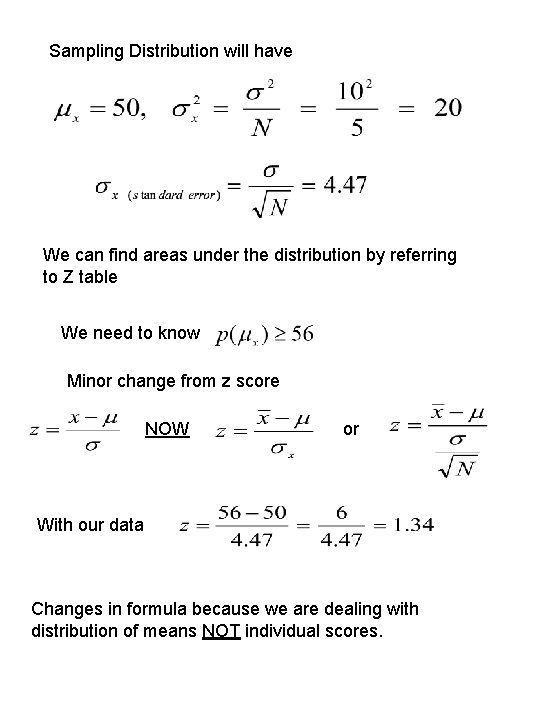Sampling Distribution will have We can find areas under the distribution by referring to Z table We need to know Minor change from z score NOW or With our data Changes in formula because we are dealing with distribution of means NOT individual scores.From Z table we find is 0. 0901 Because we want a two-tailed test we double 0. 0901 (2)0. 0901 = 0. 1802 NOT REJECT H 0 or is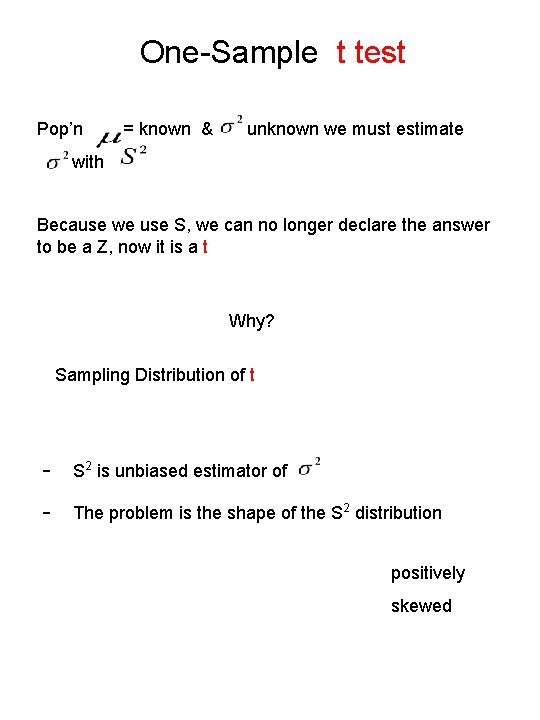One-Sample t test Pop’n = known & unknown we must estimate with Because we use S, we can no longer declare the answer to be a Z, now it is a t Why? Sampling Distribution of t - S 2 is unbiased estimator of - The problem is the shape of the S 2 distribution positively skewed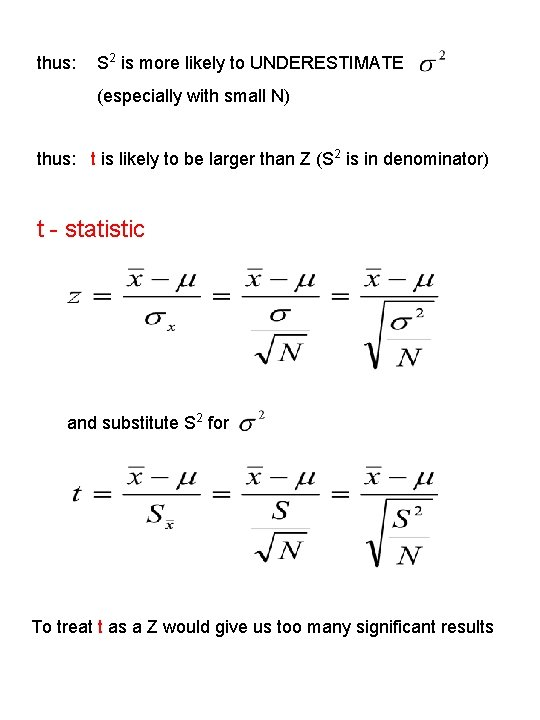thus: S 2 is more likely to UNDERESTIMATE (especially with small N) thus: t is likely to be larger than Z (S 2 is in denominator) t - statistic and substitute S 2 for To treat t as a Z would give us too many significant results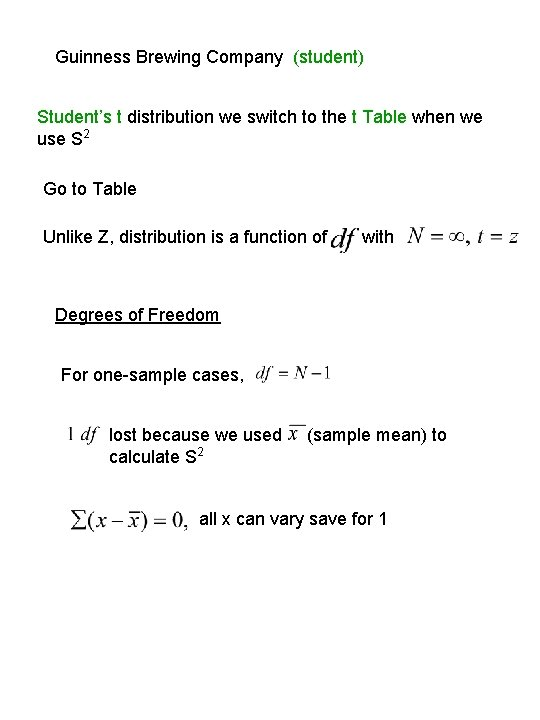Guinness Brewing Company (student) Student’s t distribution we switch to the t Table when we use S 2 Go to Table Unlike Z, distribution is a function of with Degrees of Freedom For one-sample cases, lost because we used calculate S 2 (sample mean) to all x can vary save for 1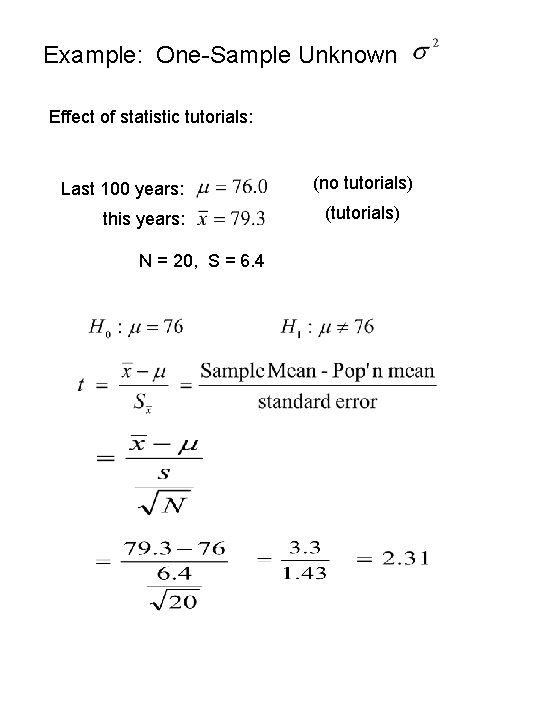Example: One-Sample Unknown Effect of statistic tutorials: Last 100 years: this years: N = 20, S = 6. 4 (no tutorials) (tutorials)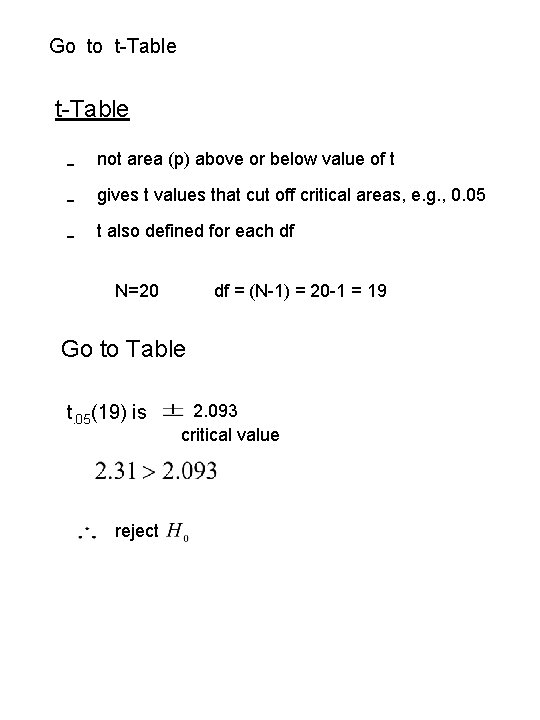Go to t-Table - not area (p) above or below value of t gives t values that cut off critical areas, e. g. , 0. 05 t also defined for each df N=20 df = (N-1) = 20 -1 = 19 Go to Table t. 05(19) is reject 2. 093 critical value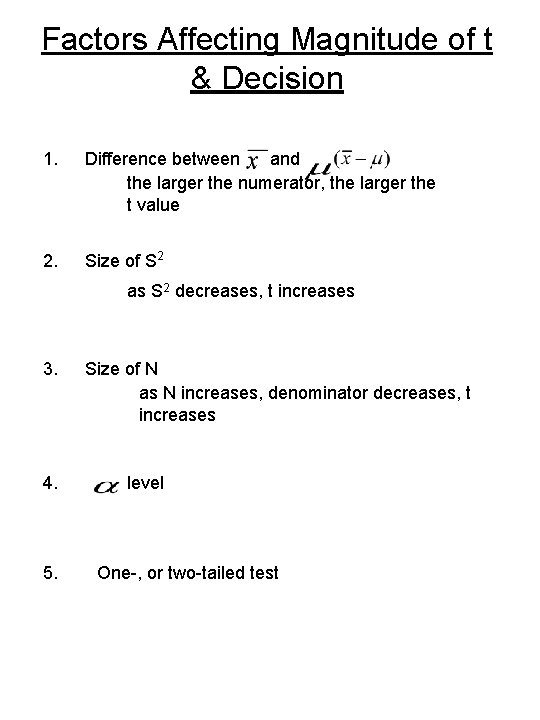Factors Affecting Magnitude of t & Decision 1. Difference between and the larger the numerator, the larger the t value 2. Size of S 2 as S 2 decreases, t increases 3. 4. 5. Size of N as N increases, denominator decreases, t increases level One-, or two-tailed test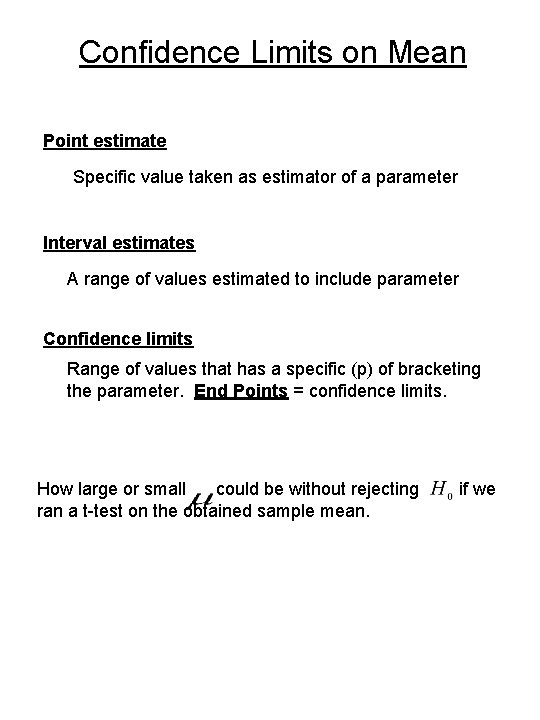Confidence Limits on Mean Point estimate Specific value taken as estimator of a parameter Interval estimates A range of values estimated to include parameter Confidence limits Range of values that has a specific (p) of bracketing the parameter. End Points = confidence limits. How large or small could be without rejecting ran a t-test on the obtained sample mean. if weConfidence Limits (C. I. ) We already know , S and We know critical value for t at We solve for Rearranging Using +2. 993 and -2. 993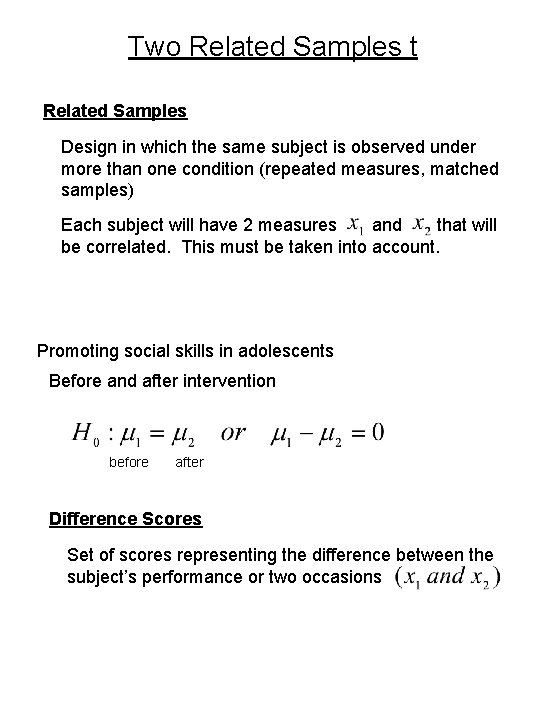Two Related Samples t Related Samples Design in which the same subject is observed under more than one condition (repeated measures, matched samples) Each subject will have 2 measures and that will be correlated. This must be taken into account. Promoting social skills in adolescents Before and after intervention before after Difference Scores Set of scores representing the difference between the subject’s performance or two occasions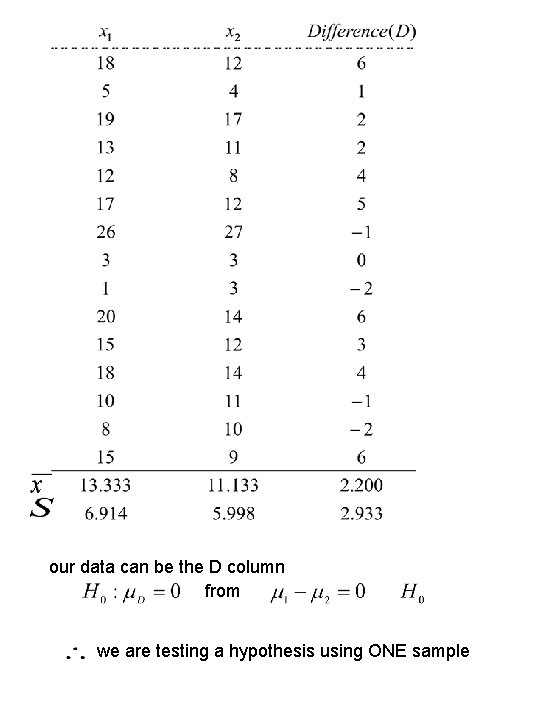our data can be the D column from we are testing a hypothesis using ONE sample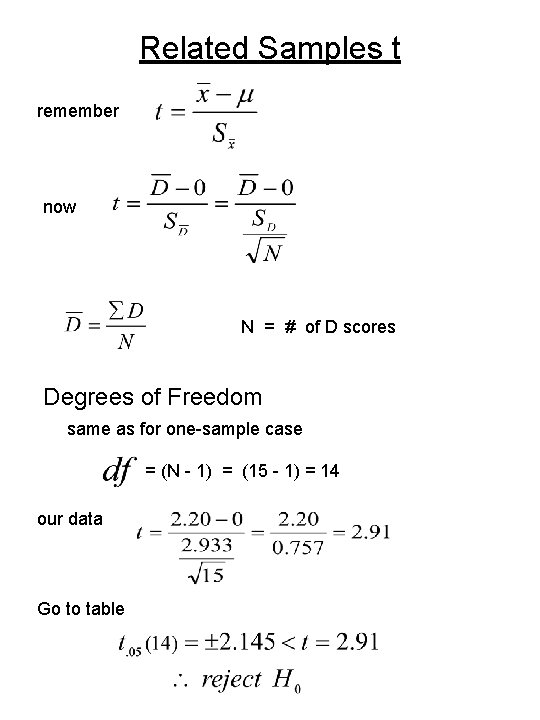Related Samples t remember now N = # of D scores Degrees of Freedom same as for one-sample case = (N - 1) = (15 - 1) = 14 our data Go to table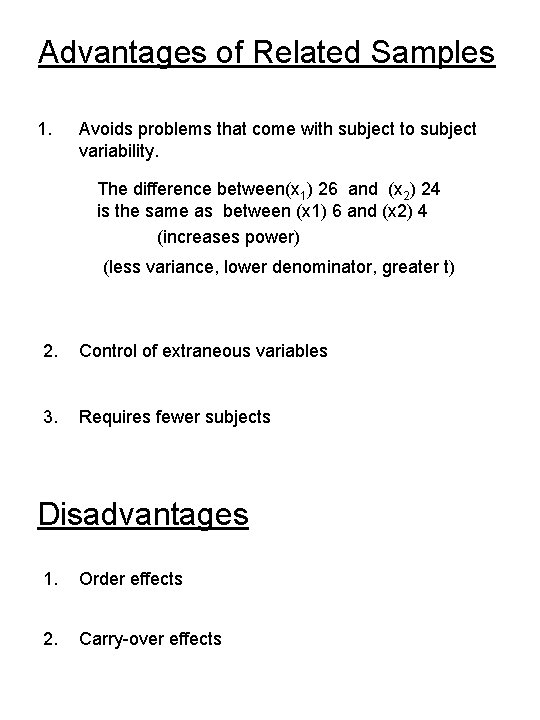Advantages of Related Samples 1. Avoids problems that come with subject to subject variability. The difference between(x 1) 26 and (x 2) 24 is the same as between (x 1) 6 and (x 2) 4 (increases power) (less variance, lower denominator, greater t) 2. Control of extraneous variables 3. Requires fewer subjects Disadvantages 1. Order effects 2. Carry-over effects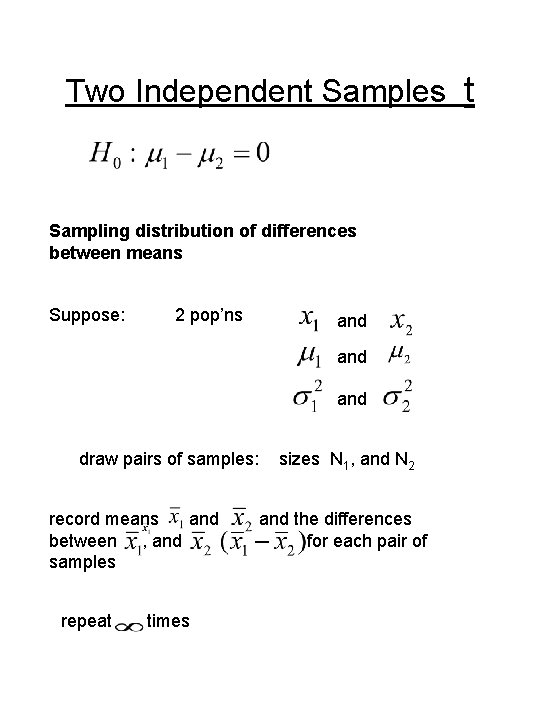Two Independent Samples t Sampling distribution of differences between means Suppose: 2 pop’ns and and draw pairs of samples: record means and between , and samples repeat times sizes N 1, and N 2 and the differences for each pair of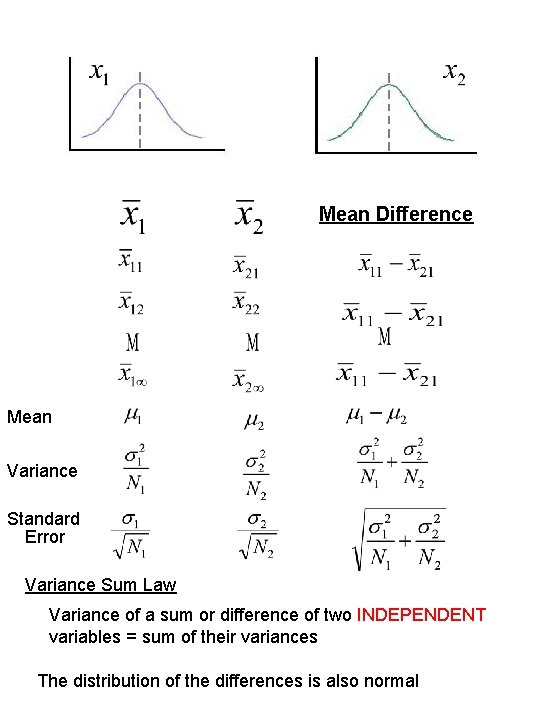Mean Difference Mean Variance Standard Error Variance Sum Law Variance of a sum or difference of two INDEPENDENT variables = sum of their variances The distribution of the differences is also normal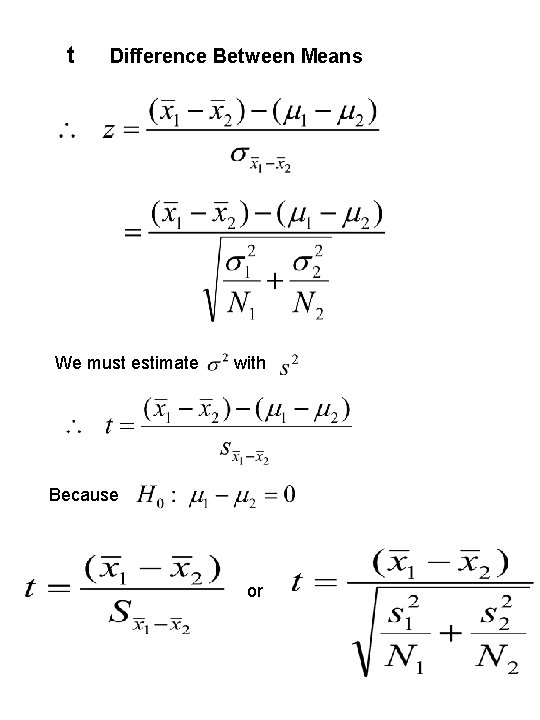t Difference Between Means We must estimate with Because or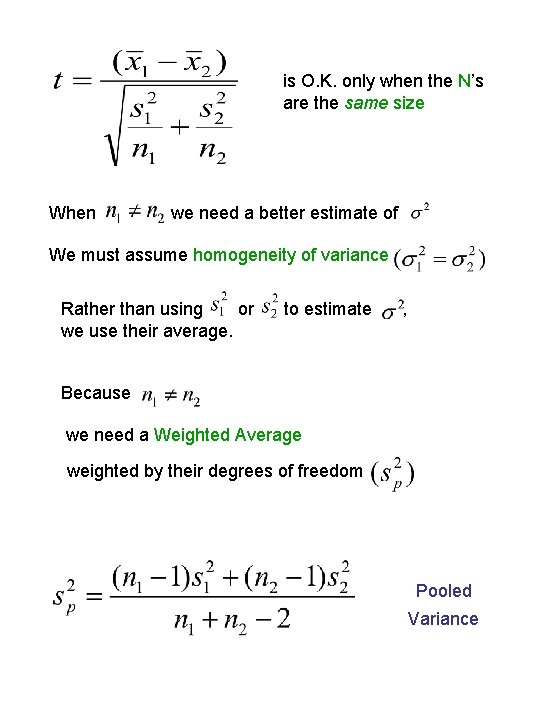is O. K. only when the N’s are the same size When we need a better estimate of We must assume homogeneity of variance Rather than using or we use their average. to estimate , Because we need a Weighted Average weighted by their degrees of freedom Pooled VarianceNow come from formula for Standard Error Degrees of Freedom two means have been used to calculate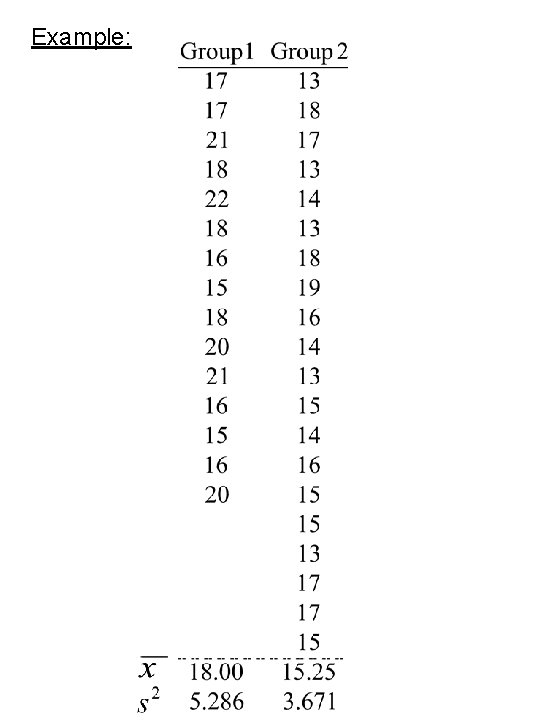Example: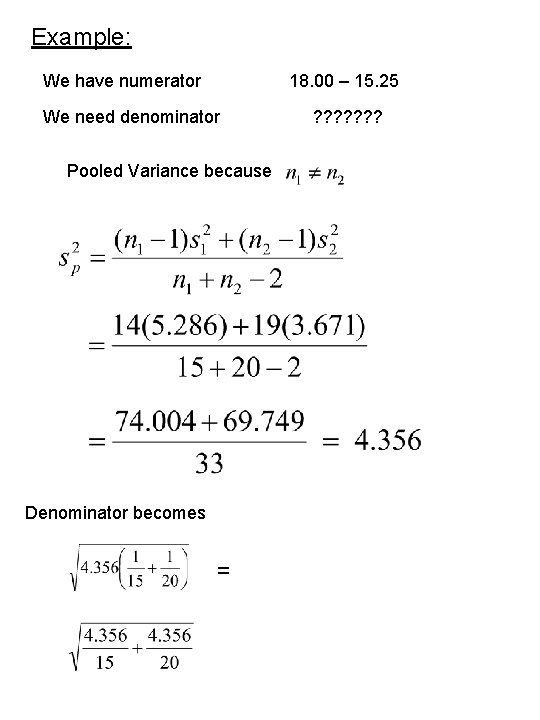Example: We have numerator 18. 00 – 15. 25 We need denominator Pooled Variance because Denominator becomes = ? ? ? ?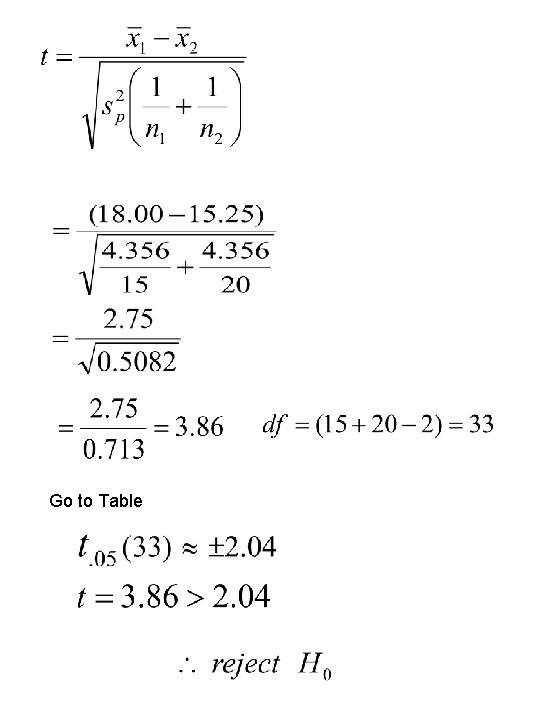Go to Table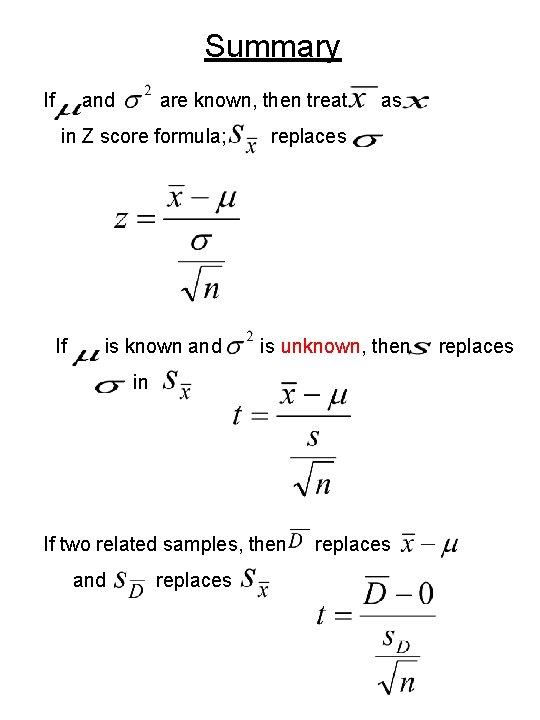Summary If and are known, then treat in Z score formula; If is known and as replaces is unknown, then in If two related samples, then and replacesIf two independent samples, and Ns are of equal size, then is replaced by If two independent samples, and Ns are NOT equal, then and are replaced by